# Trigonometric Ratios of Compound Angles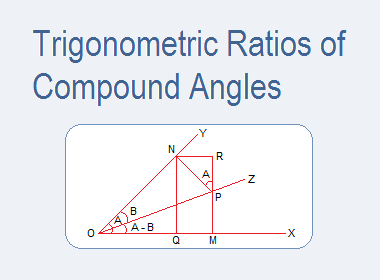## Trigonometric Ratios of Compound Angles

The sum or difference of two or more angles is said to be a compound angle. If A and B are two angles then A + B or A - B are known as the compound angles.

********************

10 Math Problems officially announces the release of Quick Math Solver, an Android App on the Google Play Store for students around the world.

********************

We express the trigonometrical ratios of compound angle A + B and A - B in terms of trigonometrical ratios of the angles A and B.The trigonometrical ratios of the angles A + B and A - B are known as the addition and subtraction formula respectively.

### Trigonometric Ratios of Compound Angle A + B (Addition Formula)

Let a revolving line start from OX and trace out an angle XOY = A and revolve further through an angle YOZ = B. Therefore, XOZ = A + B.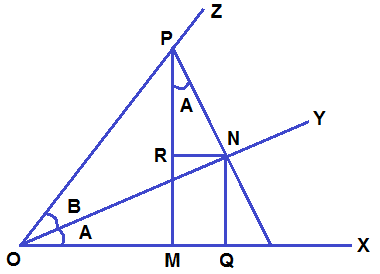Let, P be any point on OZ. Draw PM perpendicular to OX and PN perpendicular to OY. From N draw NQ perpendicular to OX and NR perpendicular to PM.

Here, RPN = 90° - PNR     [  NRMP]

= RNO   [  PNOY]

= NOQ   [  RN OX]

= A

Again, RMQN is a rectangle. So, MR = QN and RN = MQ.

Now from right angle ΔOMP,#### Tangent Formula of Compound Angle (A + B)#### Cotangent Formula of Compound Angle (A + B)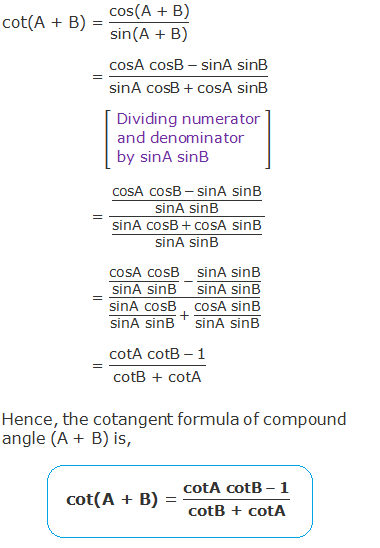### Trigonometric Ratios of Compound Angle A – B (Subtraction Formula)

Let a revolving line start from OX and trace out an angle XOY = A and then revolve back through an angle YOZ = B. Therefore, XOZ = A - B.Let, P be any point on the line OZ. Draw PM perpendicular to OX and PN perpendicular to OY. From N draw NQ perpendicular to OX and NR perpendicular to MP produced.

Here, RPN = 90° - PNR     [  PRNR]

= RNY    [  PNOY]

= XOY   [  OX NR]

= A

Again, QMRN is a rectangle. So, QN = MR and QM = NR.

Now from right angle ΔOMP,#### Tangent Formula of Compound Angle (A – B)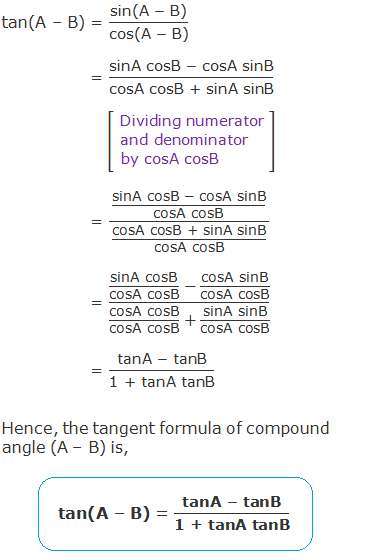#### Cotangent Formula of Compound Angle (A - B)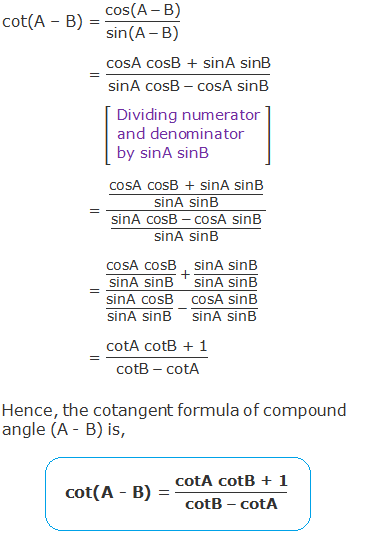### List of Trigonometric Formula for Compound Angles A + B and A – B### Worked Out Examples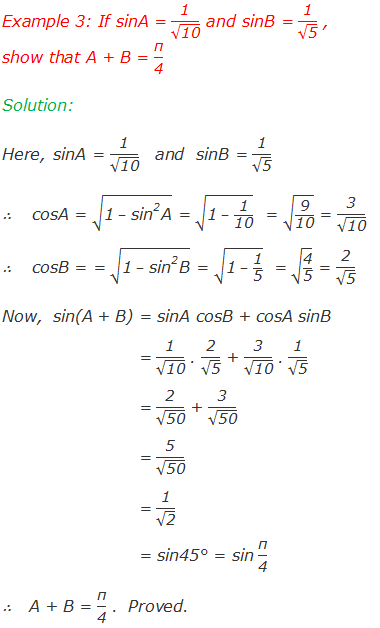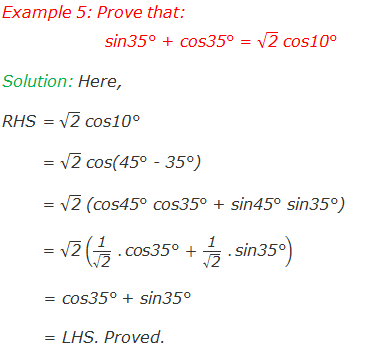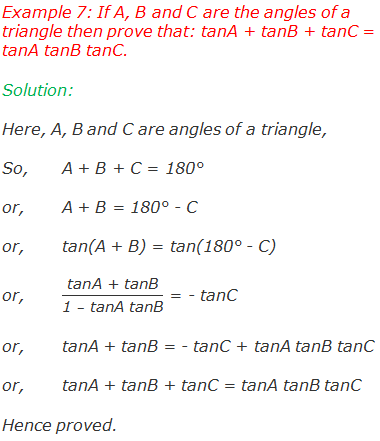#### Do you have any questions regarding the trigonometric ratios of compound angles?

You can ask your questions or problems here, in the comment section below.